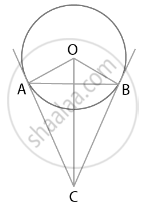# If a chord AB subtends an angle of 60° at the centre of a circle, then angle between the tangents at A and B is also 60°. - Mathematics

MCQ
True or False

If a chord AB subtends an angle of 60° at the centre of a circle, then angle between the tangents at A and B is also 60°.

• True

• False

#### Solution

This statement is False.

Explanation:

Consider the given figure. In which we have a circle with centre O and AB a chord with ∠AOB = 60°Since, tangent to any point on the circle is perpendicular to the radius through point of contact,

We get,

OA ⏊ AC and OB ⏊ CB

∠OBC = ∠OAC = 90°  .......[Equation (1)]

We get,

∠OBC + ∠OAC + ∠AOB + ∠ACB = 360°

90° + 90° + 60° + ∠ACB = 360°

∠ACB = 120°

Hence, the angle between two tangents is 120°.

Concept: Concept of Circle - Centre, Radius, Diameter, Arc, Sector, Chord, Segment, Semicircle, Circumference, Interior and Exterior, Concentric Circles
Is there an error in this question or solution?

#### APPEARS IN

NCERT Mathematics Exemplar Class 10
Chapter 9 Circles
Exercise 9.2 | Q 1 | Page 104
Share